# vs.eyeandcontacts.com

## Chapter 9 Data Handling 9.1

Question 1: In a Mathematics test, the following marks were obtained by 40 students. Arrange these marks in a table using tally marks.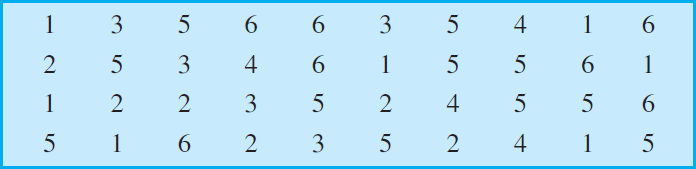a) Find how many students obtained marks equal to or more than 7.
b) How many students obtained marks below 4?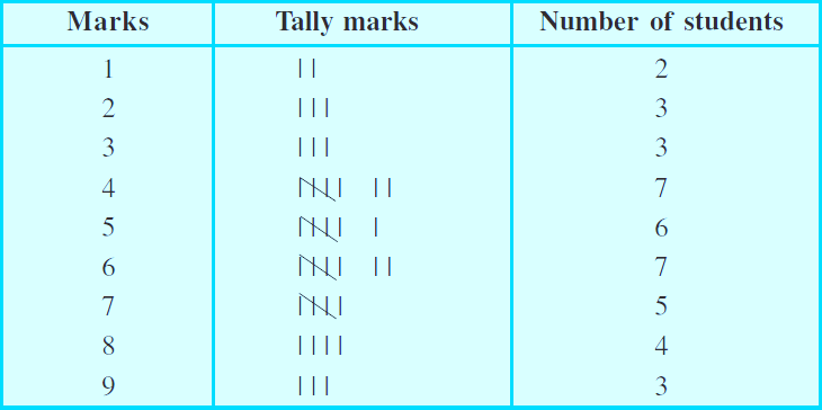a)
Students who got 7 marks = 5
Students who got 8 marks = 4
Students who got 9 marks = 3

= Students who obtained marks equal to or more than 7 = Students who got 7 marks + Students who got 8 marks + Students who got 9 marks
= 5 + 4 + 3
= 12

Therefore, 12 students got marks equal to or more than 7.

b)
Students who got 3 marks = 3
Students who got 2 marks = 3
Students who got 1 marks = 2

= Students who obtained marks less than 4 = Students who got 3 marks + Students who got 2 marks + Students who got 1 marks
= 3 + 3 + 2
= 8

Therefore, 8 students got marks less than 4.

Question 2: Following is the choice of sweets of 30 students of Class VI.
a) Arrange the names of sweets in a table using tally marks.
b) Which sweet is preferred by most of the students?

a)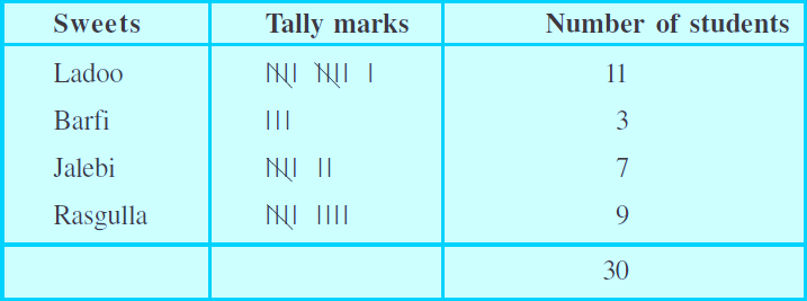b) Ladoo is the sweet which preferred by most of the students.

Question 3: Catherine threw a dice 40 times and noted the number appearing each time as shown below:Make a table and enter the data using tally marks. Find the number that appeared.
a) The minimum number of times.
b) The maximum number of times.
c) Find those numbers that appear an equal number of times.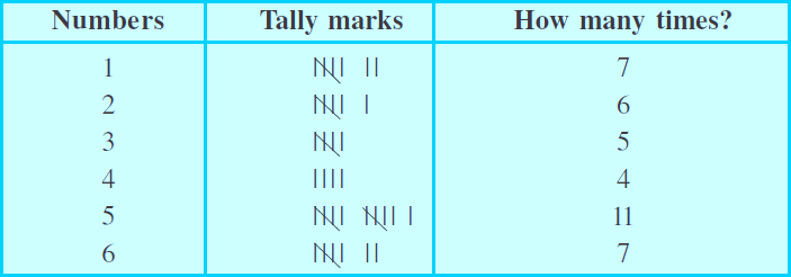a) The minimum number of times is 4.

b) The maximum number of times is 5.

c) The number which appeared an equal number of times is 1 and 6.

Question 4: Following pictograph shows the number of tractors in five villages.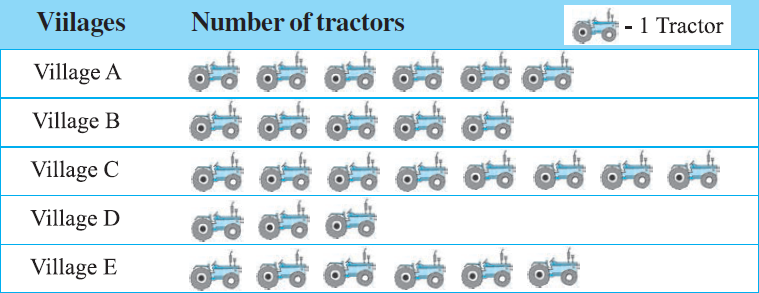Observe the pictograph and answer the following questions.
i) Which village has the minimum number of tractors?
ii) Which village has the maximum number of tractors?
iii) How many more tractors village C has as compared to village B.
iv) What is the total number of tractors in all the five villages?

i) Village D has the minimum number of tractors.

ii) Village C has the maximum number of tractors.

iii) 3 tractors village C has as compared to village B.

iv)
Tractor in village A = 6
Tractor in village B = 5
Tractor in village C = 8
Tractor in village D = 3
Tractor in village E = 6

= 6 + 5 + 8 + 3 + 6 = 28
Therefore, the total number of tractors in all the five villages is 28.

Question 5: The number of girl students in each class of a co-educational middle school is depicted by the pictograph: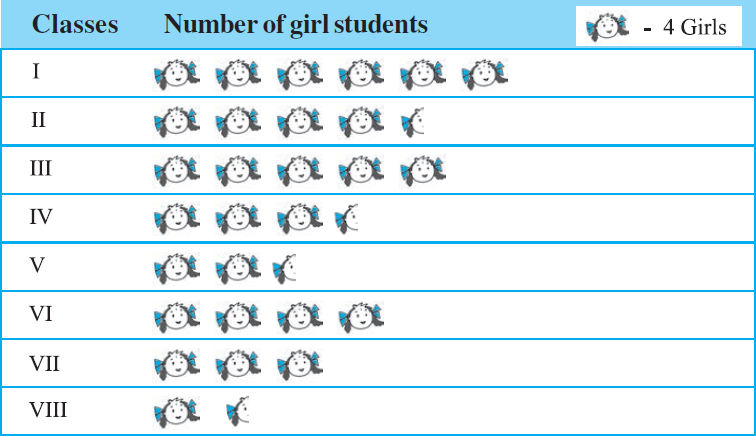Observe this pictograph and answer the following questions :
a) Which class has the minimum number of girl students?
b) Is the number of girls in Class VI less than the number of girls in Class V?
c) How many girls are there in Class VII?

a) Class VIII has the minimum number of girl students.

b) No, the number of girls in Class VI is more than the number of girls in Class V.

c) There are 12 girls in Class VII.

Question 6: The sale of electric bulbs on different days of a week is shown below: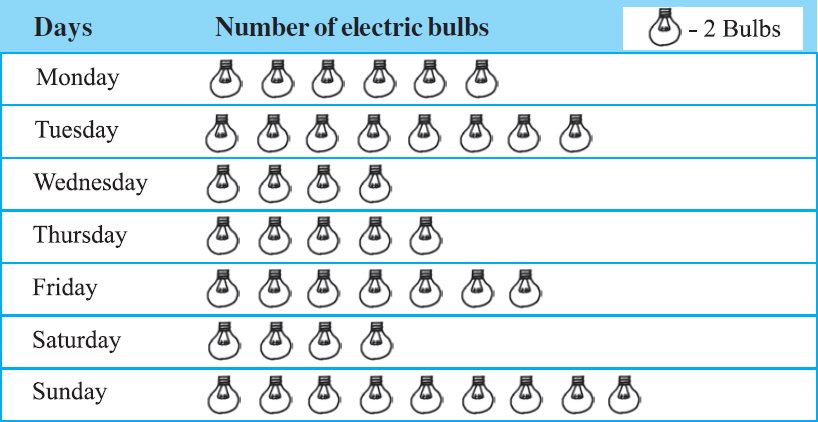Observe the pictograph and answer the following questions:
a) How many bulbs were sold on Friday?
b) On which day were the maximum number of bulbs sold?
c) On which of the days same number of bulbs were sold?
d) On which of the days minimum number of bulbs were sold?
e) If one big carton can hold 9 bulbs. How many cartons were needed in the given week?

a) 14 bulbs were sold on Friday.

b) On Sunday, the maximum number of bulbs were sold.

c) On Wednesday and Saturday, same number of bulbs were sold.

d) On Wednesday and Saturday, minimum number of bulbs were sold.

e)
Number of bulbs sold on Monday = 12
Number of bulbs sold on Tuesday = 16
Number of bulbs sold on Wednesday = 8
Number of bulbs sold on Thursday = 10
Number of bulbs sold on Friday = 14
Number of bulbs sold on Saturday = 8
Number of bulbs sold on Sunday = 18

= 12 + 16 + 8 + 10 + 14 + 8 + 18
= 86

Total bulbs = 86
Number of cartons required for 86 bulbs = ?
Number of cartons required for 9 bulbs = 1

= 86/9
= 10 cartons

Therefore, 10 cartons are required.

Question 7: In a village six fruit merchants sold the following number of fruit baskets in a particular season: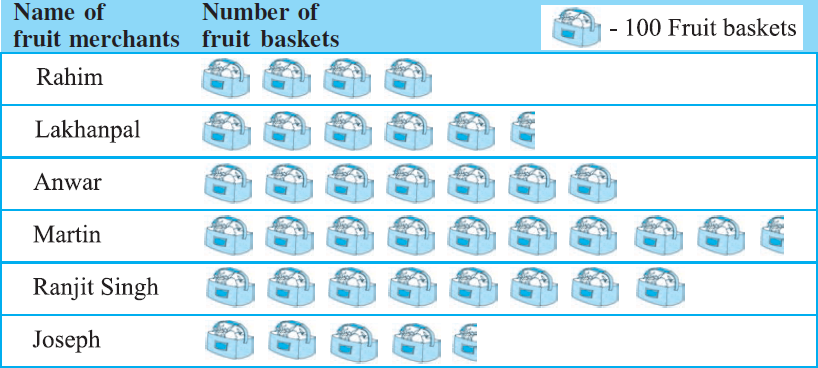Observe this pictograph and answer the following questions :
a) Which merchant sold the maximum number of baskets?
b) How many fruit baskets were sold by Anwar?
c) The merchants who have sold 600 or more number of baskets are planning to buy a godown for the next season. Can you name them?